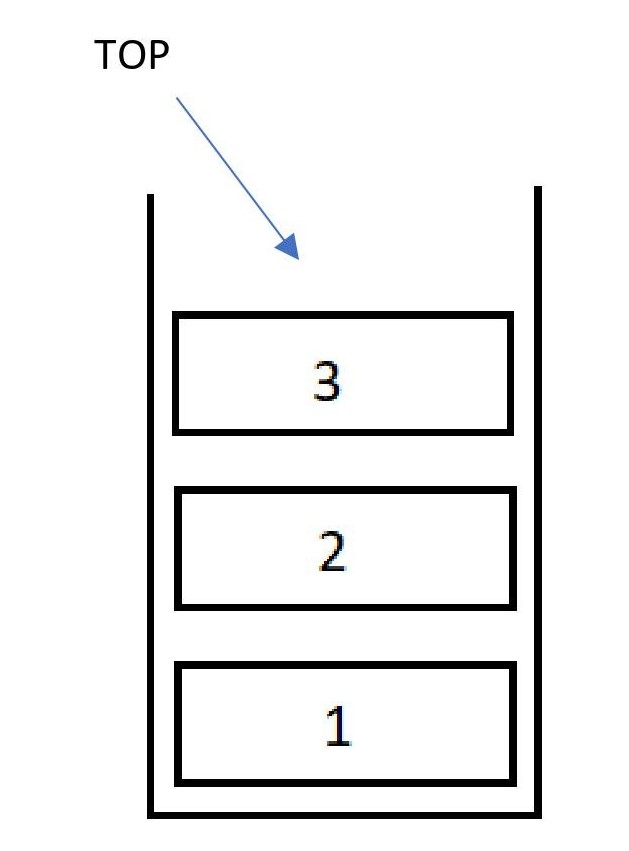×

Search anything:

# Time and Space Complexity of Stack

#### Time Complexity Algorithms Stack Data StructuresGet this book -> Problems on Array: For Interviews and Competitive Programming

In this article, we will explore about various operations on Stack Data Structure and the Time and Space Complexity of each operation for various cases like Best case, Average case and Worst case.

1. Basics of Stack
2. Push in Stack
• Time Complexity
• Space Complexity
3. POP in Stack
• Time Complexity
• Space Complexity
4. Peek in Stack
• Time Complexity
• Space Complexity
5. Conclusion

Pre-requisite:

As a summary:

Operation Best Time Complexity Worst Time Complexity Average Time Complexity Space Complexity
Push O(1) O(N) O(1) O(1)
Pop O(1) O(1) O(1) O(1)
Peek O(1) O(1) O(1) O(1)

Note the performance of both Array and Linked List based implementation of Stack is same.

# Basics of Stack

A Stack is a Linear Data Structure in which Operations are performed in a specific order known as LIFO(Last In First Out) or FILO (First In Last Out). Operations on Stack occur only at one end called as the TOP of the stack. Stack can be implemented using Arrays and LinkedLists.

Stack have many applications like:

• Conversion of infix to postfix expressions
• Forward - Backward Surfing in browsers use Stack
• Undo Mechanism uses Stacks internally.
• Stacks are used to perform recursion

Real world Stack examples would include :

• Plates on a tray
• Stack of Books
• Stack of coins etc.

Let's consider an example of stackIn this example above , we have 3 elements and the top of the stack is pointing towards the topmost element which is 3. The Top always points towards the last inserted element.

## Operations on Stacks

The most common operations that we perform on stacks are :

# Push in Stack

Push operation basically means insertion of an element into a stack , which is done through the top pointer, the top pointer points to the newest added element.

For example let's consider the stack below , the top points at 2 .

``````    |   |
|   |
| 2 |   <- Top of the Stack
|_1_|
``````

Let's Push 3 into the stack , then the stack would look like:

``````    |   |
| 3 |  <- Top of the Stack
| 2 |
|_1_|
``````

### Time Complexity

• Worst Case Scenario would be O(n) in case of a array implementation of stack where the array is completely filled, then the array size needs to be changed and all the elements must be copied from one array to another , this would result in time being O(n). In case of a LinkedList approach, time remains constant O(1).
Example:

``````  | 3 |  <- Top of the Stack
| 2 |
|_1_|
S
``````

consider the above stack S , if we had to add another element say 4 to this Stack, it would result in an overflow as the array is filled, so how do we avoid it? We can extend the array size so we can accommodate more elements into the stack

``````    |   |
|   |
| 4 |  <- Top of the Stack
| 3 |
| 2 |
|_1_|
S
``````

after extending and copying back the elements , new element can be inserted at the top of the stack.

• Best Case Scenario is O(1) as only one elements needs to be pushed onto the stack.
• Average Case Scenario would be O(1).

### Space Complexity

• Space complexity of Push Operation is O(1).

### POP in Stack

Pop operation deletes an element from the stack and returns it , the topmost element pointed by the top is deleted in Pop operation.

For example let's consider the stack below , the top points at 3 .

``````    |   |
| 3 |  <- Top of the Stack
| 2 |
|_1_|
``````

Let's Pop from the stack , then the stack would look like:

``````    |   |
|   |
| 2 |  <- Top of the Stack
|_1_|
``````

### Time Complexity

• Worst Case Scenario is O(1) , as Deletion operation only removes the top element.
• Best Case Scenario is O(1).
• Average Case Scenario would be O(1) as only the top element is needed to be removed.

### Space Complexity

• Space Complexity of Pop Operation is O(1) as no additional space is required for it.

### Peek in Stack

Peek operation returns the topmost element from the stack , no addition or deletion is involved in this operation.

For example let's consider the stack below , the top points at 3 .

``````    |   |
| 3 |  <- Top of the Stack
| 2 |
|_1_|
``````

The Peek operation would return 3 , no changes to the stack are made.

#### Time Complexity

• The Average , Worst and Best Time Complexities of Peek operation are O(1), as peeking only returns the top of the stack.

#### Space Complexity

Space Complexity of Peek Operation is O(1) as no additional space is required for it.

## Conclusion

Stack is a very useful data structure with many uses. It is an essential part of every program as all the programming languages internally use stack for function calls and many more operations. To summarize , the time and space Complexities of Stack are:

Operation Best Time Complexity Worst Time Complexity Average Time Complexity Space Complexity
Push O(1) O(n) O(1) O(1)
Pop O(1) O(1) O(1) O(1)
Peek O(1) O(1) O(1) O(1)

Note the performance of both Array and Linked List based implementation of Stack is same.

With this article at OpenGenus, you must have the complete idea of Time and Space Complexity of different Stack operations.#### Mohd Ehtesham Uddin Qureshi

Mohd Ehtesham Uddin Qureshi completed B.E in Computer Science from Muffakham Jah College of Engineering and Technology (2021). He is an Intern at OpenGenus.

Time and Space Complexity of Stack